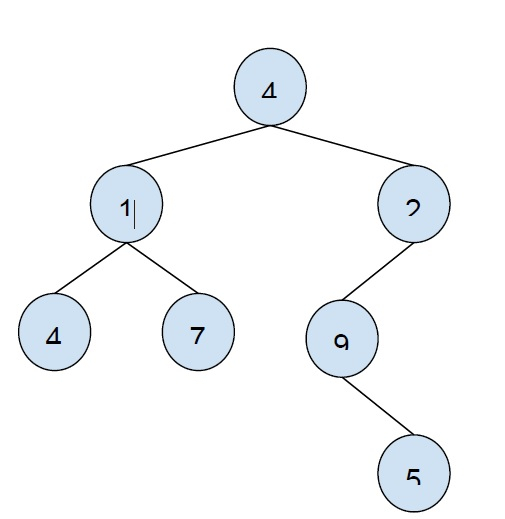# Maximum sum of non-leaf nodes among all levels of the given binary tree in C++

In this problem, we are given a binary tree. Our task is to create a program that will find the maximum sum of non-leaf nodes among all levels of the given binary tree in c++.

Problem description − we will calculate the sum of all non-leaf nodes of the tree and every level and then print the maximum sum.

Let’s take an example to understand the problem,

InputOutput − 9

Explanation − the sum of non-leaf nodes at each level −

Level 1: 4
Level 2: 1+2 = 3
Level 3: 9 (4, 7 are leaf nodes)
Level 4: 0

To solve this problem, we will have to do the level order traversal of the binary tree and find the sum of all node that are non-leaf nodes and then find the max sum of them

So, at each level, we will check if the node has left or right child, if not then add it to the sum. And maintain a maxSum that stores the maximum sum till the level. If the sum of all non-leaf nodes is greater than maxSum, then we will initialize the sum of that level to the maximum.

## Example

Program to show the implementation of our solution,

Live Demo

#include <bits/stdc++.h>
using namespace std;
struct Node {
int data;
struct Node *left, *right;
};
int maxLevelSum(struct Node* root){
if (root == NULL)
return 0;
int maxSum = root->data;
queue<Node*> q;
q.push(root);
while (!q.empty()) {
int count = q.size();
int levelSum = 0;
while (count--) {
Node* temp = q.front();
q.pop();
if (temp->left != NULL || temp->right != NULL)
levelSum = levelSum + temp->data;
if (temp->left != NULL)
q.push(temp->left);
if (temp->right != NULL)
q.push(temp->right);
}
maxSum = max(levelSum, maxSum);
}
return maxSum;
}
struct Node* insertNode(int data) {
struct Node* node = new Node;
node->data = data;
node->left = node->right = NULL;
return (node);
}
int main() {
struct Node* root = insertNode(6);
root->left = insertNode(1);
root->right = insertNode(2);
root->left->left = insertNode(4);
root->left->right = insertNode(7);
root->right->right = insertNode(9);
root->right->right->left = insertNode(5);
cout<<"The maximum sum of all non-lead nodes at a level of the binary tree is "<<maxLevelSum(root);
return 0;
}

## Output

The maximum sum of all non-lead nodes at a level of the binary tree is 9